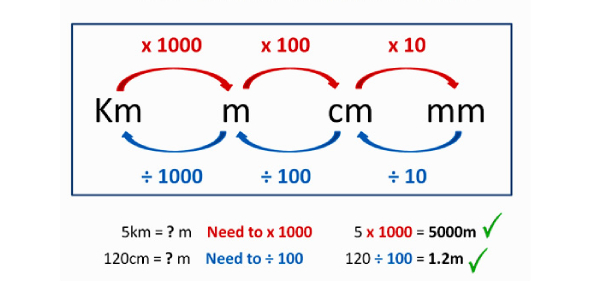# Test Your Knowledge Of Metric System With Metric Conversions Quiz!

Approved & Edited by ProProfs Editorial Team
At ProProfs Quizzes, our dedicated in-house team of experts takes pride in their work. With a sharp eye for detail, they meticulously review each quiz. This ensures that every quiz, taken by over 100 million users, meets our standards of accuracy, clarity, and engagement.
| Written by Fpaige
F
Fpaige
Community Contributor
Quizzes Created: 7 | Total Attempts: 25,724
Questions: 10 | Attempts: 15,603SettingsThe metric system is an alternative system of measurement that uses three basic units from which all others are calculated. This metric conversion quiz is aimed at gauging your knowledge of conversion. These metrics are grams for weight, meters for length, and seconds for time. Take the test below and see what you understand about the metric system and conversions with some examples. The quiz will help you learn some new facts and concepts too. All the best!

• 1.

### Use the  King  Henry slide or ladder method to solve: 15 km = ____ m

• A.

.15 m

• B.

1,500 m

• C.

.015 m

• D.

15,000 m

D. 15,000 m
Explanation
Determine how many steps and which direction to move on the slide/ladder.

Rate this question:

• 2.

### True or False? To measure the depth of the sea, use kilometers.

• A.

True

• B.

False

B. False
Explanation
The statement is false because kilometers are used to measure distance, not depth. The depth of the sea is typically measured in units such as meters or feet.

Rate this question:

• 3.

### What is the basic unit in weight?

• A.

Grams

• B.

Kilograms

• C.

Pounds

• D.

None of the above

A. Grams
Explanation
The basic unit of weight is grams. Grams are a metric unit of measurement commonly used to measure the weight of small objects. It is the smallest unit of weight in the metric system and is often used in scientific and everyday measurements. Kilograms and pounds are larger units of weight, while "None of the above" is not correct as grams is indeed the basic unit of weight.

Rate this question:

• 4.

### Use the  King  Henry slide or ladder method to solve: 209 g = ___________ kg

• A.

.209 kg

• B.

2,090 kg

• C.

209,000 kg

• D.

2.09 kg

A. .209 kg
Explanation
Determine how many steps and which direction to move on the slide/ladder.

Rate this question:

• 5.

### Use the  King  Henry slide or ladder method to solve: 8.3 m = _________  cm

• A.

.830 cm

• B.

830 cm

• C.

8,300 cm

• D.

83 cm

B. 830 cm
Explanation
Determine how many steps and which direction to move on the slide/ladder.

Rate this question:

• 6.

### True or False: The metric volume of a liquid is measured in Liters.

• A.

True

• B.

False

A. True
Explanation
The metric volume of a liquid is measured in liters. This is because the liter is the standard unit of measurement for volume in the metric system. It is commonly used to measure the volume of liquids, such as water, milk, or juice. One liter is equal to 1000 milliliters, and it is a larger unit compared to milliliters or cubic centimeters. Therefore, it is correct to say that the metric volume of a liquid is measured in liters.

Rate this question:

• 7.

### It would make sense to measure your desk using :

• A.

Kilometers

• B.

Millimeters

• C.

Centimeters

• D.

Meters

C. Centimeters
Explanation
Measuring a desk using centimeters would make sense because centimeters are a more appropriate unit of measurement for smaller objects like desks. Kilometers would be too large of a unit, millimeters would be too small and not practical, and meters could be used but centimeters provide a more precise measurement for a desk.

Rate this question:

• 8.

### Use the King Henry slide or ladder method to solve: 1000 mg = _____________ g

• A.

.01 g

• B.

1 g

• C.

100 g

• D.

10 g

B. 1 g
Explanation
Determine how many steps and which direction to move on the slide/ladder.

Rate this question:

• 9.

### The metric system is based on units of powers of

• A.

10

• B.

6

• C.

2

• D.

8

A. 10
Explanation
The metric system is based on units of powers of 10. This means that each unit is a multiple or fraction of 10. For example, the meter is the base unit for length and is equal to 10 decimeters, 100 centimeters, or 1000 millimeters. This system allows for easy conversion between different units by simply moving the decimal point. It is widely used around the world for its simplicity and consistency.

Rate this question:

• 10.

### Use the  King  Henry slide or ladder method to solve: 180 cm = _________________ mm

• A.

180 mm

• B.

18 mm

• C.

.180 mm

• D.

1,800 mmBack to top